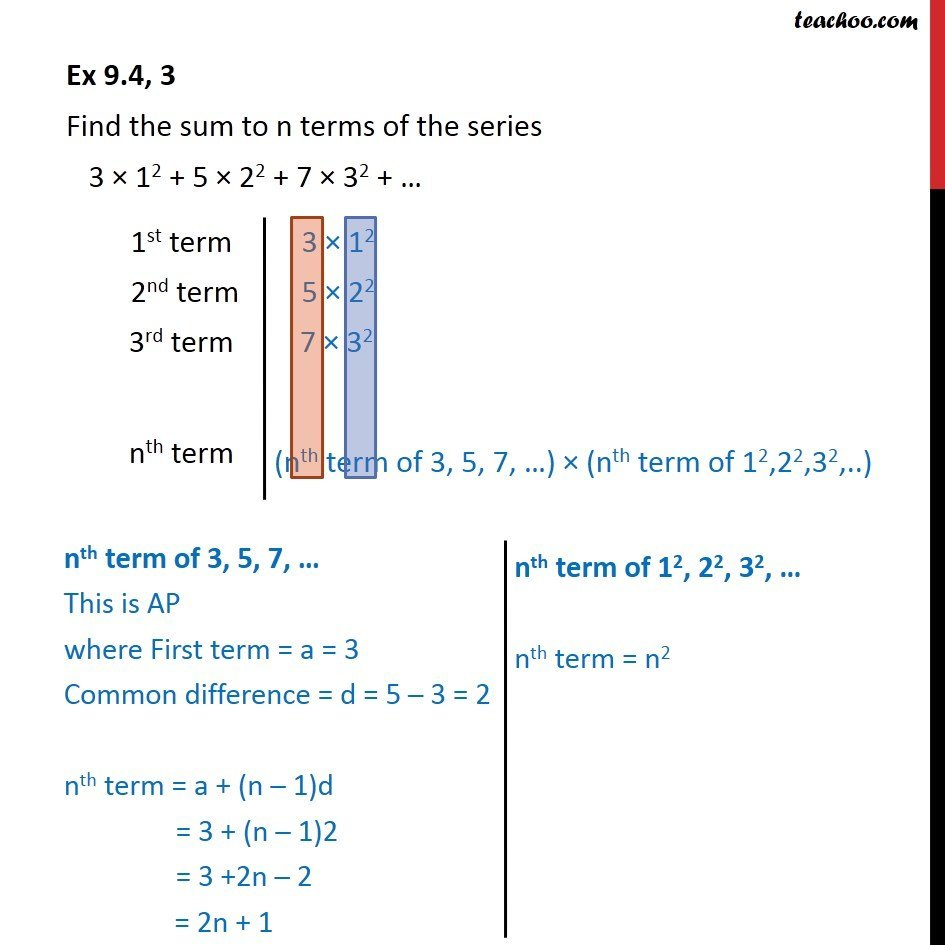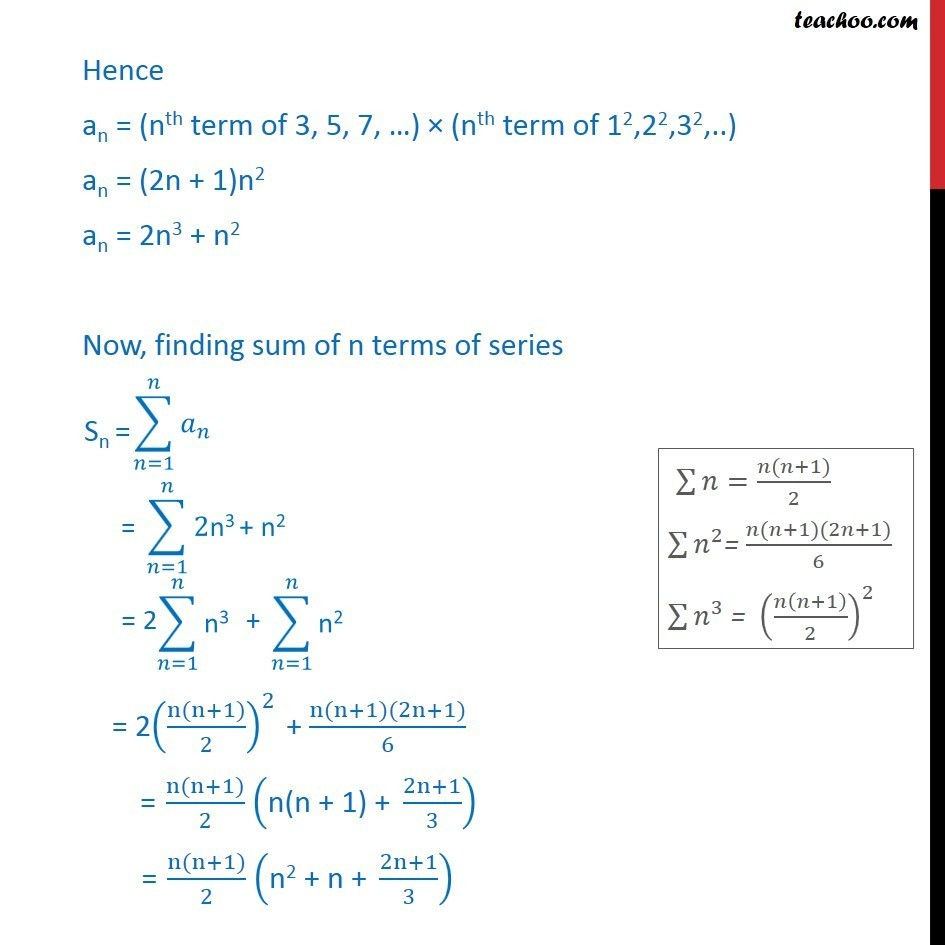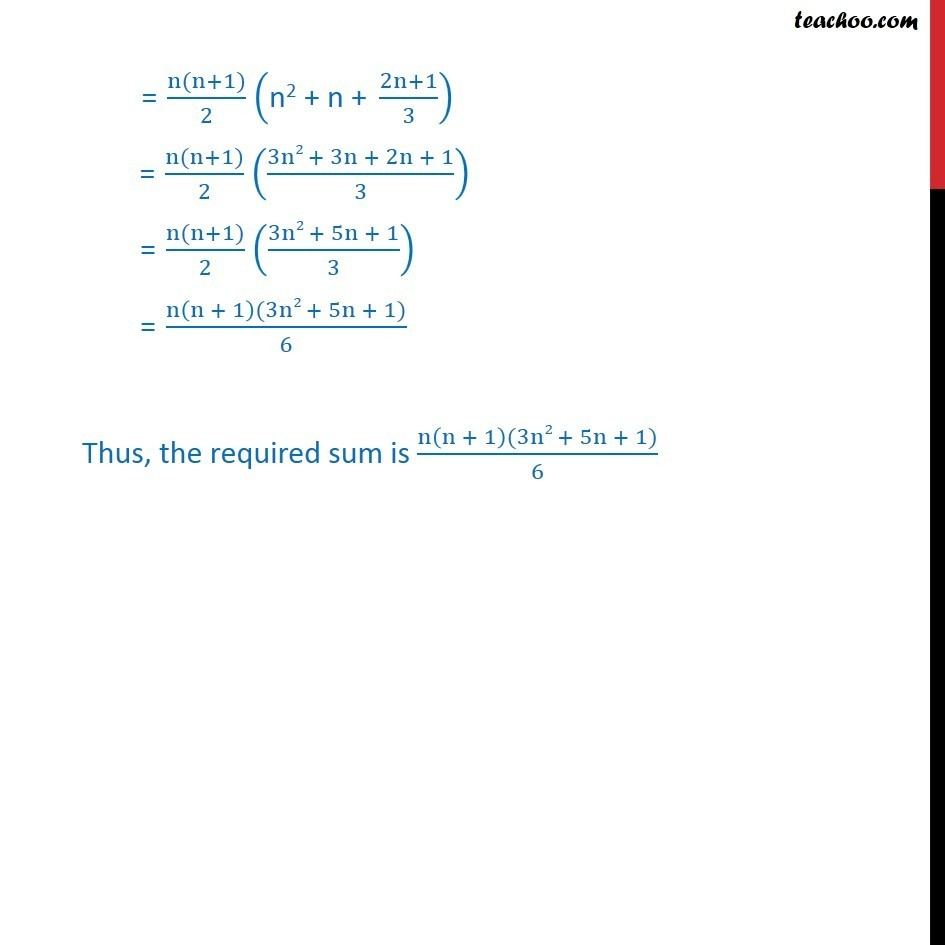Ex 9.4

Chapter 9 Class 11 Sequences and Series
Serial order wiseGet live Maths 1-on-1 Classs - Class 6 to 12

### Transcript

Ex 9.4, 3 Find the sum to n terms of the series 3 12 + 5 22 + 7 32 + Hence an = (nth term of 3, 5, 7, ) (nth term of 12,22,32,..) an = (2n + 1)n2 an = 2n3 + n2 Now, finding sum of n terms of series = 2((n(n+1))/2)^2 + (n(n+1)(2n+1))/6 = (n(n+1))/2 ("n(n + 1) + " (2n+1)/3) = (n(n+1))/2 ("n2 + n + " (2n+1)/3) = (n(n+1))/2 ("n2 + n + " (2n+1)/3) = (n(n+1))/2 ((3n2 + 3n + 2n + 1)/3) = (n(n+1))/2 ((3n2 + 5n + 1)/3) = (n(n + 1)(3n2 + 5n + 1))/6 Thus, the required sum is (n(n + 1)(3n2 + 5n + 1))/6